Confidence Intervals

# 40 A Confidence Interval for a Population Standard Deviation Unknown, Small Sample Case

In practice, we rarely know the population standard deviation. In the past, when the sample size was large, this did not present a problem to statisticians. They used the sample standard deviation s as an estimate for σ and proceeded as before to calculate a confidence interval with close enough results. This is what we did in (Figure) above. The point estimate for the standard deviation, s, was substituted in the formula for the confidence interval for the population standard deviation. In this case there 80 observation well above the suggested 30 observations to eliminate any bias from a small sample. However, statisticians ran into problems when the sample size was small. A small sample size caused inaccuracies in the confidence interval.

William S. Goset (1876–1937) of the Guinness brewery in Dublin, Ireland ran into this problem. His experiments with hops and barley produced very few samples. Just replacing σ with s did not produce accurate results when he tried to calculate a confidence interval. He realized that he could not use a normal distribution for the calculation; he found that the actual distribution depends on the sample size. This problem led him to “discover” what is called the Student’s t-distribution. The name comes from the fact that Gosset wrote under the pen name “A Student.”

Up until the mid-1970s, some statisticians used the normal distribution approximation for large sample sizes and used the Student’s t-distribution only for sample sizes of at most 30 observations.

If you draw a simple random sample of size n from a population with mean μ and unknown population standard deviation σ and calculate the t-score t =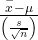, then the t-scores follow a Student’s t-distribution with n – 1 degrees of freedom. The t-score has the same interpretation as the z-score. It measures how far in standard deviation units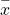is from its mean μ. For each sample size n, there is a different Student’s t-distribution.

The degrees of freedom, n – 1, come from the calculation of the sample standard deviation s. Remember when we first calculated a sample standard deviation we divided the sum of the squared deviations by n − 1, but we used n deviations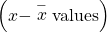to calculate s. Because the sum of the deviations is zero, we can find the last deviation once we know the other n – 1 deviations. The other n – 1 deviations can change or vary freely. We call the number n – 1 the degrees of freedom (df) in recognition that one is lost in the calculations. The effect of losing a degree of freedom is that the t-value increases and the confidence interval increases in width.

Properties of the Student’s t-Distribution
• The graph for the Student’s t-distribution is similar to the standard normal curve and at infinite degrees of freedom it is the normal distribution. You can confirm this by reading the bottom line at infinite degrees of freedom for a familiar level of confidence, e.g. at column 0.05, 95% level of confidence, we find the t-value of 1.96 at infinite degrees of freedom.
• The mean for the Student’s t-distribution is zero and the distribution is symmetric about zero, again like the standard normal distribution.
• The Student’s t-distribution has more probability in its tails than the standard normal distribution because the spread of the t-distribution is greater than the spread of the standard normal. So the graph of the Student’s t-distribution will be thicker in the tails and shorter in the center than the graph of the standard normal distribution.
• The exact shape of the Student’s t-distribution depends on the degrees of freedom. As the degrees of freedom increases, the graph of Student’s t-distribution becomes more like the graph of the standard normal distribution.
• The underlying population of individual observations is assumed to be normally distributed with unknown population mean μ and unknown population standard deviation σ. This assumption comes from the Central Limit theorem because the individual observations in this case are the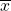s of the sampling distribution. The size of the underlying population is generally not relevant unless it is very small. If it is normal then the assumption is met and doesn’t need discussion.

A probability table for the Student’s t-distribution is used to calculate t-values at various commonly-used levels of confidence. The table gives t-scores that correspond to the confidence level (column) and degrees of freedom (row). When using a t-table, note that some tables are formatted to show the confidence level in the column headings, while the column headings in some tables may show only corresponding area in one or both tails. Notice that at the bottom the table will show the t-value for infinite degrees of freedom. Mathematically, as the degrees of freedom increase, the t distribution approaches the standard normal distribution. You can find familiar Z-values by looking in the relevant alpha column and reading value in the last row.

A Student’s t table (See (Figure)) gives t-scores given the degrees of freedom and the right-tailed probability.

The Student’s t distribution has one of the most desirable properties of the normal: it is symmetrical. What the Student’s t distribution does is spread out the horizontal axis so it takes a larger number of standard deviations to capture the same amount of probability. In reality there are an infinite number of Student’s t distributions, one for each adjustment to the sample size. As the sample size increases, the Student’s t distribution become more and more like the normal distribution. When the sample size reaches 30 the normal distribution is usually substituted for the Student’s t because they are so much alike. This relationship between the Student’s t distribution and the normal distribution is shown in (Figure).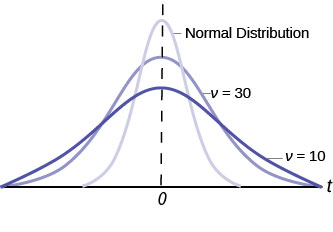This is another example of one distribution limiting another one, in this case the normal distribution is the limiting distribution of the Student’s t when the degrees of freedom in the Student’s t approaches infinity. This conclusion comes directly from the derivation of the Student’s t distribution by Mr. Gosset. He recognized the problem as having few observations and no estimate of the population standard deviation. He was substituting the sample standard deviation and getting volatile results. He therefore created the Student’s t distribution as a ratio of the normal distribution and Chi squared distribution. The Chi squared distribution is itself a ratio of two variances, in this case the sample variance and the unknown population variance. The Student’s t distribution thus is tied to the normal distribution, but has degrees of freedom that come from those of the Chi squared distribution. The algebraic solution demonstrates this result.

Development of Student’s t-distribution:
1.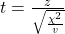Where Z is the standard normal distribution and χ2 is the chi-squared distribution with v degrees of freedom.

2.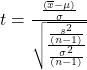by substitution, and thus Student’s t with v = n − 1 degrees of freedom is:

3.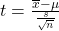Restating the formula for a confidence interval for the mean for cases when the sample size is smaller than 30 and we do not know the population standard deviation, σ: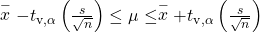Here the point estimate of the population standard deviation, s has been substituted for the population standard deviation, σ, and tν,α has been substituted for Zα. The Greek letter ν (pronounced nu) is placed in the general formula in recognition that there are many Student tv distributions, one for each sample size. ν is the symbol for the degrees of freedom of the distribution and depends on the size of the sample. Often df is used to abbreviate degrees of freedom. For this type of problem, the degrees of freedom is ν = n-1, where n is the sample size. To look up a probability in the Student’s t table we have to know the degrees of freedom in the problem.

The average earnings per share (EPS) for 10 industrial stocks randomly selected from those listed on the Dow-Jones Industrial Average was found to be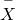= 1.85 with a standard deviation of s=0.395. Calculate a 99% confidence interval for the average EPS of all the industrials listed on the DJIA.To help visualize the process of calculating a confident interval we draw the appropriate distribution for the problem. In this case this is the Student’s t because we do not know the population standard deviation and the sample is small, less than 30.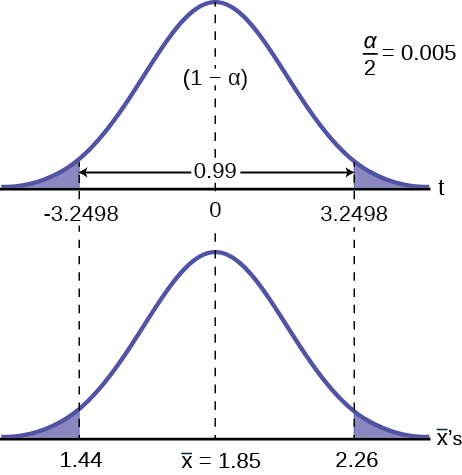To find the appropriate t-value requires two pieces of information, the level of confidence desired and the degrees of freedom. The question asked for a 99% confidence level. On the graph this is shown where (1-α) , the level of confidence , is in the unshaded area. The tails, thus, have .005 probability each, α/2. The degrees of freedom for this type of problem is n-1= 9. From the Student’s t table, at the row marked 9 and column marked .005, is the number of standard deviations to capture 99% of the probability, 3.2498. These are then placed on the graph remembering that the Student’s t is symmetrical and so the t-value is both plus or minus on each side of the mean.

Inserting these values into the formula gives the result. These values can be placed on the graph to see the relationship between the distribution of the sample means,‘s and the Student’s t distribution.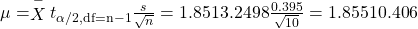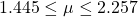We state the formal conclusion as :

With 99% confidence level, the average EPS of all the industries listed at DJIA is from ?1.44 to ?2.26.

Try It

You do a study of hypnotherapy to determine how effective it is in increasing the number of hours of sleep subjects get each night. You measure hours of sleep for 12 subjects with the following results. Construct a 95% confidence interval for the mean number of hours slept for the population (assumed normal) from which you took the data.

8.2; 9.1; 7.7; 8.6; 6.9; 11.2; 10.1; 9.9; 8.9; 9.2; 7.5; 10.5

(8.1634, 9.8032)

### References

“America’s Best Small Companies.” Forbes, 2013. Available online at http://www.forbes.com/best-small-companies/list/ (accessed July 2, 2013).

Data from Microsoft Bookshelf.

Data from http://www.forbes.com/.

“Disclosure Data Catalog: Leadership PAC and Sponsors Report, 2012.” Federal Election Commission. Available online at http://www.fec.gov/data/index.jsp (accessed July 2,2013).

“Human Toxome Project: Mapping the Pollution in People.” Environmental Working Group. Available online at http://www.ewg.org/sites/humantoxome/participants/participant-group.php?group=in+utero%2Fnewborn (accessed July 2, 2013).

### Chapter Review

In many cases, the researcher does not know the population standard deviation, σ, of the measure being studied. In these cases, it is common to use the sample standard deviation, s, as an estimate of σ. The normal distribution creates accurate confidence intervals when σ is known, but it is not as accurate when s is used as an estimate. In this case, the Student’s t-distribution is much better. Define a t-score using the following formula: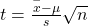The t-score follows the Student’s t-distribution with n – 1 degrees of freedom. The confidence interval under this distribution is calculated with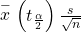where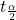is the t-score with area to the right equal to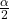, s is the sample standard deviation, and n is the sample size. Use a table, calculator, or computer to findfor a given α.

### Formula Review

s = the standard deviation of sample values.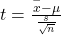is the formula for the t-score which measures how far away a measure is from the population mean in the Student’s t-distribution

df = n – 1; the degrees of freedom for a Student’s t-distribution where n represents the size of the sample

T~tdf the random variable, T, has a Student’s t-distribution with df degrees of freedom

The general form for a confidence interval for a single mean, population standard deviation unknown, and sample size less than 30 Student’s t is given by:Use the following information to answer the next five exercises. A hospital is trying to cut down on emergency room wait times. It is interested in the amount of time patients must wait before being called back to be examined. An investigation committee randomly surveyed 70 patients. The sample mean was 1.5 hours with a sample standard deviation of 0.5 hours.

Identify the following:

1.=_______
2.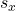=_______
3. n =_______
4. n – 1 =_______

Define the random variables X andin words.

X is the number of hours a patient waits in the emergency room before being called back to be examined.is the mean wait time of 70 patients in the emergency room.

Which distribution should you use for this problem?

Construct a 95% confidence interval for the population mean time spent waiting. State the confidence interval, sketch the graph, and calculate the error bound.

CI: (1.3808, 1.6192)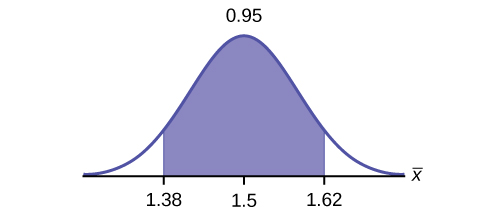EBM = 0.12

Explain in complete sentences what the confidence interval means.

Use the following information to answer the next six exercises: One hundred eight Americans were surveyed to determine the number of hours they spend watching television each month. It was revealed that they watched an average of 151 hours each month with a standard deviation of 32 hours. Assume that the underlying population distribution is normal.

Identify the following:

1.=_______
2.=_______
3. n =_______
4. n – 1 =_______
1.= 151
2.= 32
3. n = 108
4. n – 1 = 107

Define the random variable X in words.

Define the random variablein words.is the mean number of hours spent watching television per month from a sample of 108 Americans.

Which distribution should you use for this problem?

Construct a 99% confidence interval for the population mean hours spent watching television per month. (a) State the confidence interval, (b) sketch the graph, and (c) calculate the error bound.

CI: (142.92, 159.08)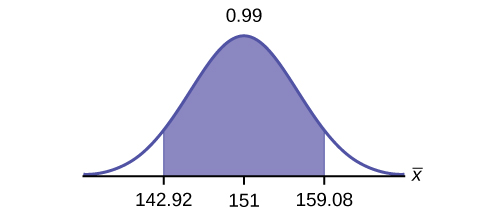EBM = 8.08

Why would the error bound change if the confidence level were lowered to 95%?

Use the following information to answer the next 13 exercises: The data in (Figure) are the result of a random survey of 39 national flags (with replacement between picks) from various countries. We are interested in finding a confidence interval for the true mean number of colors on a national flag. Let X = the number of colors on a national flag.

X Freq.
1 1
2 7
3 18
4 7
5 6

Calculate the following:

1.=______
2.=______
3. n =______
1. 3.26
2. 1.02
3. 39

Define the random variablein words.

What isestimating?

μ

Is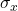known?

As a result of your answer to (Figure), state the exact distribution to use when calculating the confidence interval.

t38

Construct a 95% confidence interval for the true mean number of colors on national flags.

How much area is in both tails (combined)?

How much area is in each tail?

0.025

Calculate the following:

1. lower limit
2. upper limit
3. error bound

The 95% confidence interval is_____.

(2.93, 3.59)

Fill in the blanks on the graph with the areas, the upper and lower limits of the Confidence Interval and the sample mean.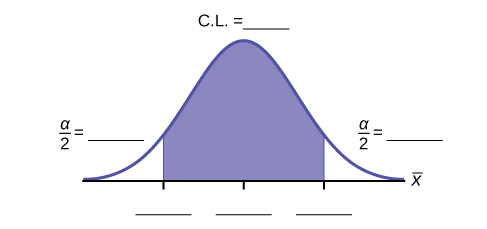In one complete sentence, explain what the interval means.

We are 95% confident that the true mean number of colors for national flags is between 2.93 colors and 3.59 colors.

Using the same,, and level of confidence, suppose that n were 69 instead of 39. Would the error bound become larger or smaller? How do you know?

The error bound would become EBM = 0.245. This error bound decreases because as sample sizes increase, variability decreases and we need less interval length to capture the true mean.

Using the same,, and n = 39, how would the error bound change if the confidence level were reduced to 90%? Why?

### Homework

In six packages of “The Flintstones® Real Fruit Snacks” there were five Bam-Bam snack pieces. The total number of snack pieces in the six bags was 68. We wish to calculate a 96% confidence interval for the population proportion of Bam-Bam snack pieces.

1. Define the random variables X and P′ in words.
2. Which distribution should you use for this problem? Explain your choice
3. Calculate p′.
4. Construct a 96% confidence interval for the population proportion of Bam-Bam snack pieces per bag.
1. State the confidence interval.
2. Sketch the graph.
3. Calculate the error bound.
5. Do you think that six packages of fruit snacks yield enough data to give accurate results? Why or why not?

A random survey of enrollment at 35 community colleges across the United States yielded the following figures: 6,414; 1,550; 2,109; 9,350; 21,828; 4,300; 5,944; 5,722; 2,825; 2,044; 5,481; 5,200; 5,853; 2,750; 10,012; 6,357; 27,000; 9,414; 7,681; 3,200; 17,500; 9,200; 7,380; 18,314; 6,557; 13,713; 17,768; 7,493; 2,771; 2,861; 1,263; 7,285; 28,165; 5,080; 11,622. Assume the underlying population is normal.

1.= __________
2.= __________
3. n = __________
4. n – 1 = __________
1. Define the random variables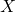andin words.
2. Which distribution should you use for this problem? Explain your choice.
3. Construct a 95% confidence interval for the population mean enrollment at community colleges in the United States.
1. State the confidence interval.
2. Sketch the graph.
4. What will happen to the error bound and confidence interval if 500 community colleges were surveyed? Why?
1. 8629
2. 6944
3. 35
4. 34
1.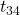1. CI: (6244, 11,014)
2.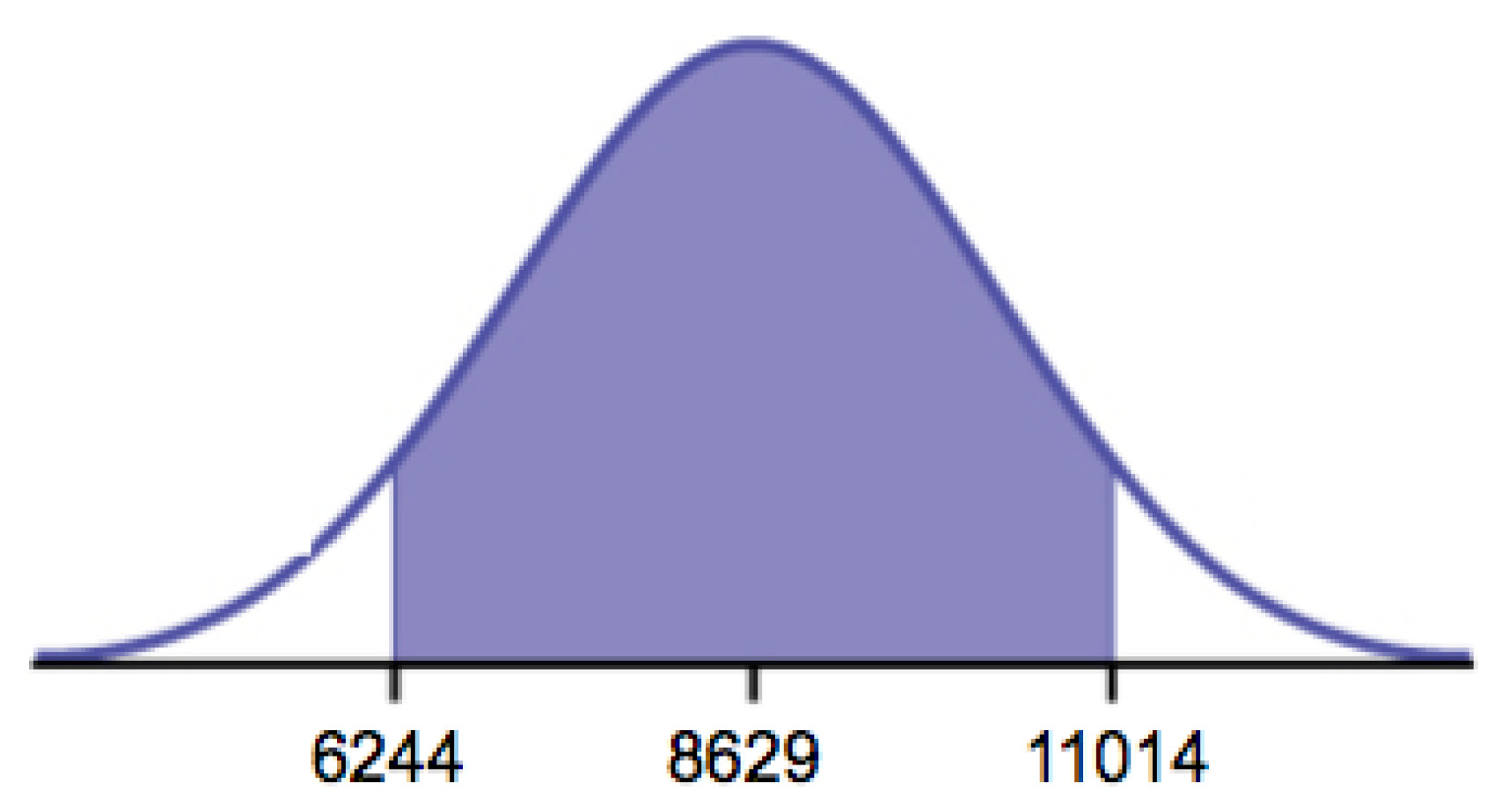2. It will become smaller

Suppose that a committee is studying whether or not there is waste of time in our judicial system. It is interested in the mean amount of time individuals waste at the courthouse waiting to be called for jury duty. The committee randomly surveyed 81 people who recently served as jurors. The sample mean wait time was eight hours with a sample standard deviation of four hours.

1.= __________
2.= __________
3. n = __________
4. n – 1 = __________
1. Define the random variablesandin words.
2. Which distribution should you use for this problem? Explain your choice.
3. Construct a 95% confidence interval for the population mean time wasted.
1. State the confidence interval.
2. Sketch the graph.
4. Explain in a complete sentence what the confidence interval means.

A pharmaceutical company makes tranquilizers. It is assumed that the distribution for the length of time they last is approximately normal. Researchers in a hospital used the drug on a random sample of nine patients. The effective period of the tranquilizer for each patient (in hours) was as follows: 2.7; 2.8; 3.0; 2.3; 2.3; 2.2; 2.8; 2.1; and 2.4.

1.= __________
2.= __________
3. n = __________
4. n – 1 = __________
1. Define the random variablein words.
2. Define the random variablein words.
3. Which distribution should you use for this problem? Explain your choice.
4. Construct a 95% confidence interval for the population mean length of time.
1. State the confidence interval.
2. Sketch the graph.
5. What does it mean to be “95% confident” in this problem?
1.= 2.51
2.= 0.318
3. n = 9
4. n – 1 = 8
1. the effective length of time for a tranquilizer
2. the mean effective length of time of tranquilizers from a sample of nine patients
3. We need to use a Student’s-t distribution, because we do not know the population standard deviation.
1. CI: (2.27, 2.76)
2. Check student’s solution.
4. If we were to sample many groups of nine patients, 95% of the samples would contain the true population mean length of time.

Suppose that 14 children, who were learning to ride two-wheel bikes, were surveyed to determine how long they had to use training wheels. It was revealed that they used them an average of six months with a sample standard deviation of three months. Assume that the underlying population distribution is normal.

1.= __________
2.= __________
3. n = __________
4. n – 1 = __________
1. Define the random variablein words.
2. Define the random variablein words.
3. Which distribution should you use for this problem? Explain your choice.
4. Construct a 99% confidence interval for the population mean length of time using training wheels.
1. State the confidence interval.
2. Sketch the graph.
5. Why would the error bound change if the confidence level were lowered to 90%?

The Federal Election Commission (FEC) collects information about campaign contributions and disbursements for candidates and political committees each election cycle. A political action committee (PAC) is a committee formed to raise money for candidates and campaigns. A Leadership PAC is a PAC formed by a federal politician (senator or representative) to raise money to help other candidates’ campaigns.

The FEC has reported financial information for 556 Leadership PACs that operating during the 2011–2012 election cycle. The following table shows the total receipts during this cycle for a random selection of 30 Leadership PACs.

 ?46,500.00 ?0 ?40,966.50 ?105,887.20 ?5,175.00 ?29,050.00 ?19,500.00 ?181,557.20 ?31,500.00 ?149,970.80 ?2,555,363.20 ?12,025.00 ?409,000.00 ?60,521.70 ?18,000.00 ?61,810.20 ?76,530.80 ?119,459.20 ?0 ?63,520.00 ?6,500.00 ?502,578.00 ?705,061.10 ?708,258.90 ?135,810.00 ?2,000.00 ?2,000.00 ?0 ?1,287,933.80 ?219,148.30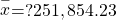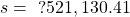Use this sample data to construct a 95% confidence interval for the mean amount of money raised by all Leadership PACs during the 2011–2012 election cycle. Use the Student’s t-distribution.Note that we are not given the population standard deviation, only the standard deviation of the sample.

There are 30 measures in the sample, so n = 30, and df = 30 – 1 = 29

CL = 0.96, so α = 1 – CL = 1 – 0.96 = 0.04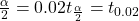= 2.150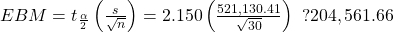EBM = ?251,854.23 – ?204,561.66 = ?47,292.57+ EBM = ?251,854.23+ ?204,561.66 = ?456,415.89

We estimate with 96% confidence that the mean amount of money raised by all Leadership PACs during the 2011–2012 election cycle lies between ?47,292.57 and ?456,415.89.

Forbes magazine published data on the best small firms in 2012. These were firms that had been publicly traded for at least a year, have a stock price of at least ?5 per share, and have reported annual revenue between ?5 million and ?1 billion. The (Figure) shows the ages of the corporate CEOs for a random sample of these firms.

 48 58 51 61 56 59 74 63 53 50 59 60 60 57 46 55 63 57 47 55 57 43 61 62 49 67 67 55 55 49

Use this sample data to construct a 90% confidence interval for the mean age of CEO’s for these top small firms. Use the Student’s t-distribution.

Unoccupied seats on flights cause airlines to lose revenue. Suppose a large airline wants to estimate its mean number of unoccupied seats per flight over the past year. To accomplish this, the records of 225 flights are randomly selected and the number of unoccupied seats is noted for each of the sampled flights. The sample mean is 11.6 seats and the sample standard deviation is 4.1 seats.

1.= __________
2.= __________
3. n = __________
4. n-1 = __________
1. Define the random variablesandin words.
2. Which distribution should you use for this problem? Explain your choice.
3. Construct a 92% confidence interval for the population mean number of unoccupied seats per flight.
1. State the confidence interval.
2. Sketch the graph.
1.= 11.6
2.= 4.1
3. n = 225
4. n – 1 = 224
1. X is the number of unoccupied seats on a single flight.is the mean number of unoccupied seats from a sample of 225 flights.
2. We will use a Student’s-t distribution, because we do not know the population standard deviation.
1. CI: (11.12 , 12.08)
2. Check student’s solution.

In a recent sample of 84 used car sales costs, the sample mean was ?6,425 with a standard deviation of ?3,156. Assume the underlying distribution is approximately normal.

1. Which distribution should you use for this problem? Explain your choice.
2. Define the random variablein words.
3. Construct a 95% confidence interval for the population mean cost of a used car.
1. State the confidence interval.
2. Sketch the graph.
4. Explain what a “95% confidence interval” means for this study.

Six different national brands of chocolate chip cookies were randomly selected at the supermarket. The grams of fat per serving are as follows: 8; 8; 10; 7; 9; 9. Assume the underlying distribution is approximately normal.

1. Construct a 90% confidence interval for the population mean grams of fat per serving of chocolate chip cookies sold in supermarkets.
1. State the confidence interval.
2. Sketch the graph.
2. If you wanted a smaller error bound while keeping the same level of confidence, what should have been changed in the study before it was done?
3. Go to the store and record the grams of fat per serving of six brands of chocolate chip cookies.
4. Calculate the mean.
5. Is the mean within the interval you calculated in part a? Did you expect it to be? Why or why not?
1. CI: (7.64 , 9.36)
2.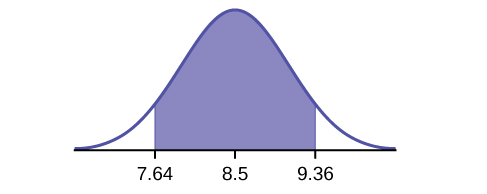1. The sample should have been increased.

A survey of the mean number of cents off that coupons give was conducted by randomly surveying one coupon per page from the coupon sections of a recent San Jose Mercury News. The following data were collected: 20¢; 75¢; 50¢; 65¢; 30¢; 55¢; 40¢; 40¢; 30¢; 55¢; ?1.50; 40¢; 65¢; 40¢. Assume the underlying distribution is approximately normal.

1.= __________
2.= __________
3. n = __________
4. n-1 = __________
1. Define the random variablesandin words.
2. Which distribution should you use for this problem? Explain your choice.
3. Construct a 95% confidence interval for the population mean worth of coupons.
1. State the confidence interval.
2. Sketch the graph.
4. If many random samples were taken of size 14, what percent of the confidence intervals constructed should contain the population mean worth of coupons? Explain why.

Use the following information to answer the next two exercises: A quality control specialist for a restaurant chain takes a random sample of size 12 to check the amount of soda served in the 16 oz. serving size. The sample mean is 13.30 with a sample standard deviation of 1.55. Assume the underlying population is normally distributed.

Find the 95% Confidence Interval for the true population mean for the amount of soda served.

1. (12.42, 14.18)
2. (12.32, 14.29)
3. (12.50, 14.10)
4. Impossible to determine

b

### Key Terms

Degrees of Freedom (df)
the number of objects in a sample that are free to vary
Normal Distribution
a continuous random variable (RV) with pdf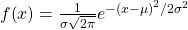, where μ is the mean of the distribution and σ is the standard deviation, notation: X ~ N(μ,σ). If μ = 0 and σ = 1, the RV is called the standard normal distribution.
Standard Deviation
a number that is equal to the square root of the variance and measures how far data values are from their mean; notation: s for sample standard deviation and σ for population standard deviation
Student’s t-Distribution
investigated and reported by William S. Gossett in 1908 and published under the pseudonym Student; the major characteristics of this random variable (RV) are:

• It is continuous and assumes any real values.
• The pdf is symmetrical about its mean of zero.
• It approaches the standard normal distribution as n get larger.
• There is a “family of t–distributions: each representative of the family is completely defined by the number of degrees of freedom, which depends upon the application for which the t is being used.